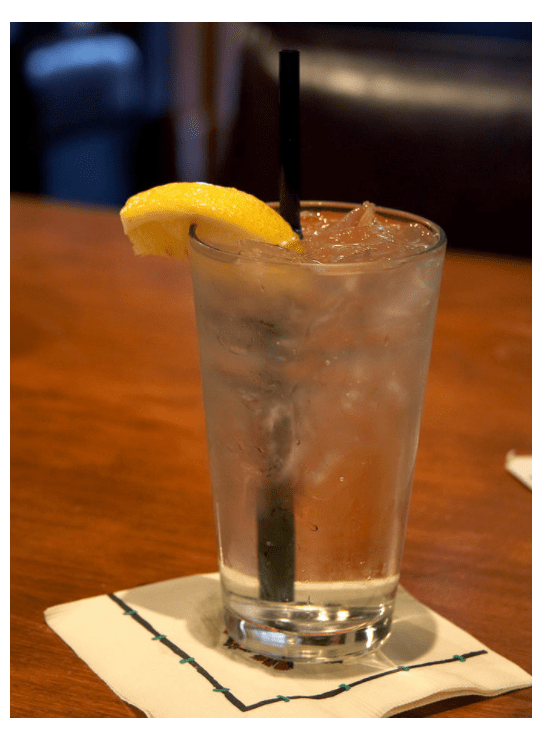Cyber Sale Extended 3 Days: ALL MCAT Products & Admissions Services

MCAT Content / Energy Changes In Chemical Reactions / Second Law Concept Of Entropy

### Second Law: Concept of Entropy

Topic: Energy Changes In Chemical Reactions

The Second Law of Thermodynamics states that the entropy (or disorder) of a system is constantly increasing. The unit of entropy is Joules per Kelvin or J/K.

Entropy is the measure of the disorder of a system and can is the energy of a system over its temperature, represented as J/K.The concept of disorder can best be described in terms of the states of matter. Solids, liquids, and gasses all have different degrees of disorder. We can think of the degrees of the number of different states the molecules in each can occupy. Because molecules in gases are able to change both volume and shape, they can be considered the most disordered. Liquids can be considered the second most disordered, and solids can be considered the least disordered.

When considering whether reactions have an increase or decrease in entropy, two factors are important: The number of moles created and states of the reactants versus the products. If a substance goes from a liquid to a solid, the molecules in the system occupy a lower number of states (think about the decrease of molecule activity in water when it freezes to ice) and therefore the entropy decreases if a substance goes from a solid to a liquid, the entropy increases.Example of increasing entropy: Ice melting in a warm room is a common example of increasing entropy.

If there are 2 moles of reactant forming 1 mole of product, the number of states possible decreases and entropy decreases, likewise, if 1 mole of reactant forms 2 moles of product, the entropy increases.

Entropy can be calculated using the equation

ΔS = q / ΔT

Where q is the heat transferred in joules and ΔT is the change in temperature in Kelvin.

Practice Questions

MCAT Official Prep (AAMC)

Chemistry Question Pack Passage 8 Question 45

Section Bank C/P Section Passage 6 Question 42

Key Points

• Entropy is represented by Joules per Kelvin or J/K.

• The entropy of a gas>liquid>solid.

• The forming more moles of a product than reactant increases entropy

Key Terms

entropy: a thermodynamic property that is the measure of a system’s thermal energy per unit temperature that is unavailable for doing useful work.

joules: unit of energy

kelvin: unit of temperature

disorder: the distance and irregularity in a system when examining the particles

Billing Information